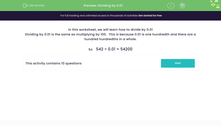# Divide by 0.01

In this worksheet, students will divide numbers by 0.01.Key stage:  KS 3

Curriculum topic:   Number

Curriculum subtopic:   Use Four Operations for All Numbers

Popular topics:   Multiplication worksheets, Division worksheets

Difficulty level:#### Worksheet Overview

In this activity, we will learn how to divide by 0.01

Dividing by 0.01 is the same as multiplying by 100.  This is because 0.01 is one hundredth and there are a hundred hundredths in a whole.

So,   542 ÷ 0.01 = 54,200  and also  542 x 100 = 54,200

Let's try another one.

What is 264 ÷ 0.01?

It is the same as multiplying 264 by 100, so the answer is 26,400

What is 4.72 ÷ 0.01?

It is the same as multiplying 4.72 by 100, so it is 472.

Does this make sense?Let's have a go at some questions now.

### What is EdPlace?

We're your National Curriculum aligned online education content provider helping each child succeed in English, maths and science from year 1 to GCSE. With an EdPlace account you’ll be able to track and measure progress, helping each child achieve their best. We build confidence and attainment by personalising each child’s learning at a level that suits them.

Get started